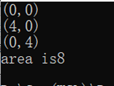# 为什么需要友元

## get/set Method

get 方法和 set 方法，是常见的获取和设置数据成员的方式。比如游戏角色遭到对方攻击后血量减少，就需要用到 set 方法，而要实时的显示就需要 set 方法。

``````#include <iostream>

using namespace std;

class Sprite    //设置角色类
{
public:
Sprite(int lb = 100) :_lifeBlood(lb) {}  //构造角色血量
int getLiseBlood()     //返回当前血量
{
return _lifeBlood;
}

void setLiseBlood(int lb)    //设置遭到攻击之后的血量
{
_lifeBlood = lb;
}

private:
int _lifeBlood;      //定义角色血量

};

void fight(Sprite & sp)  //参加战斗
{
sp.setLiseBlood(sp.getLiseBlood() - 20);    //修改变化之后的剩余血量
cout << sp.getLiseBlood() << endl;    //输出血量

}
int main()
{
Sprite sp;
fight(sp);
return 0;
}
``````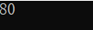``````void fight(Sprite & sp)  //参加战斗
{
//sp.setLiseBlood(sp.getLiseBlood() - 20);    //修改变化之后的剩余血量
//cout << sp.getLiseBlood() << endl;    //输出血量

//提高效率的方法
sp._lifeBlood = sp._lifeBlood - 20;
cout << sp.getLiseBlood() << endl;

}
``````

fight 全局接口，通过战斗来参加或是减少血量，对于这种频繁的操作，可以每次不通过对象，而直接操作。

``````#include <iostream>

using namespace std;

class Sprite    //设置角色类
{
friend void fight(Sprite& sp);   //将函数 fight 设置为类 Sprite 的友元
public:
Sprite(int lb = 100) :_lifeBlood(lb) {}  //构造角色血量
int getLiseBlood()     //返回当前血量
{
return _lifeBlood;
}

void setLiseBlood(int lb)    //设置遭到攻击之后的血量
{
_lifeBlood = lb;
}

private:
int _lifeBlood;      //定义角色血量

};

void fight(Sprite & sp)  //参加战斗
{
//sp.setLiseBlood(sp.getLiseBlood() - 20);    //修改变化之后的剩余血量
//cout << sp.getLiseBlood() << endl;    //输出血量

//提高效率的方法
sp._lifeBlood = sp._lifeBlood - 30;
cout << sp.getLiseBlood() << endl;

}
int main()
{
Sprite sp;
fight(sp);
return 0;
}
``````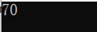get方法和set方法是标准封装的结果，但是上面使用友元会破环这种封装。

# 关系辨别

## 同类间无私处

``````mystring::mystring(const mystring & other)
{
int len = strlen(other._str);
this->_str = new char[len+1];
strcpy(this->_str,other._str);
}
``````

## 异类间有友元

struct 对象其成员默认是 public 的，所以 operator+函数中是可以成立的，但是 struct换为 class 后，其成员默认是 private 的，就会编译出错。

``````#include <iostream>

using namespace std;

struct Comlpex
{
double real;
double image;
};

Comlpex operator+(Comlpex& c1, Comlpex& c2)
{
Comlpex tmp;
tmp.real = c1.real + c2.real;
tmp.image = c1.image + c2.image;
return tmp;
}

int main()
{
Comlpex c1 = { 1,2 }, c2 = { 3,4 };
Comlpex sum = { 0,0 };
sum = c1 + c2;
return 0;
}
``````

``````#include <iostream>

using namespace std;

class Comlpex
{
friend  Comlpex operator+(Comlpex& c1, Comlpex& c2);
public:
Comlpex(double r = 0, double i = 0):real(r), image(i) {}
void DumpFromat()
{
cout << "(" << real << "," << image << ")" << endl;
}

private:
double real;
double image;
};

Comlpex operator+(Comlpex& c1, Comlpex& c2)
{
Comlpex tmp;
tmp.real = c1.real + c2.real;
tmp.image = c1.image + c2.image;
return tmp;
}

int main()
{
Comlpex c1(1,2), c2(3,4);
Comlpex sum = { 0,0 };
sum = c1 + c2;
sum.DumpFromat();
return 0;
}
``````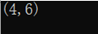# 友元函数

## 全局函数作友元

### 语法格式

``````class A
{
friend void globalfunction(A &,...)
public:
....
}
``````

### 实战操练：求两点之间的距离

``````#include<iostream>
#include<cmath>
using namespace std;
class Point
{
public:
Point(double x,double y)
{
_x = x;
_y = y;
}
void getFormatxy();
friend double distance(Point &a, Point &b);
private:
double _x, _y;
};
void Point::getFormatxy()
{
cout <<"(" << _x << "," << _y << ")" <<endl;
}
double distance(Point &a, Point &b)
{
double dx = a._x - b._x;
double dy = a._y - b._y;
return sqrt(dx*dx + dy*dy);
}
int main(void)
{
Point p1(3.0,4.0), p2(6.0,8.0);
p1.getFormatxy();
p2.getFormatxy();
double d = distance(p1, p2);
cout<< "distance is " << d <<endl;
return 0;
}
``````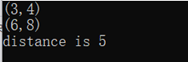## 类的成员函数作友元

### 语法格式

``````class A
{
friend void One::globalfunction(A&,...);
public:
...
}
``````

### 实战操练：求两点之间的距离

``````#include<iostream>
#include<cmath>
using namespace std;
class Point;
class ManagerPoint
{
public:
double distance(Point &a, Point &b);
};
class Point
{
public:
Point(double x, double y)
{
_x = x;
_y = y;
}
void getFormatxy();
friend double ManagerPoint::distance(Point &a, Point &b);
private:
double _x, _y;
};
void Point::getFormatxy()
{
cout << "(" << _x << "," << _y << ")" << endl;
}
double ManagerPoint::distance(Point &a, Point &b)
{ double dx = a._x - b._x;
double dy = a._y - b._y;
return sqrt(dx*dx + dy*dy);
}
int main(void)
{
Point p1(3.0, 4.0), p2(6.0, 8.0);
p1.getFormatxy();
p2.getFormatxy();
ManagerPoint mp;
float d = mp.distance(p1,p2);
cout << "distance is" << d<< endl;
return 0;
}
``````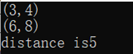## 前向声明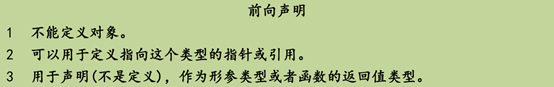# 友元类

## 声明

``````class A
{
friend class B;
public:
private:
…
};
``````

## 应用：求两点之间的距离

``````#include<iostream>
#include<cmath>
using namespace std;
class Point
{
friend class ManagerPoint;
public:
Point(double x, double y)
{
_x = x;
_y = y;
}
void getFormatxy();
private:
double _x, _y;
};
void Point::getFormatxy()
{
cout << "(" << _x << "," << _y << ")" << endl;
}
class ManagerPoint
{
public:
double distance(Point& a, Point& b);
};
double ManagerPoint::distance(Point& a, Point& b)
{
double dx = a._x - b._x;
double dy = a._y - b._y;
return sqrt(dx * dx + dy * dy);
}
int main(void)
{
Point p1(3.0, 4.0), p2(6.0, 8.0);
p1.getFormatxy();
p2.getFormatxy();
ManagerPoint mp;
float d = mp.distance(p1, p2);
cout << "distance is" << d << endl;
return 0;
}
``````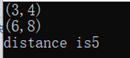## 应用：求三点构成的面积

``````#include<iostream>
#include<cmath>

using namespace std;

class Point
{
friend class ManagerPoint;
public:
Point(double x, double y)
{
_x = x;
_y = y;
}
void getFormatxy();
private:
double _x, _y;
};
void Point::getFormatxy()
{
cout << "(" << _x << "," << _y << ")" << endl;
}

class ManagerPoint
{
public:
double distance(const Point& a, const Point& b);
double getArea(const Point& a, const Point& b, const Point& c);
};

double ManagerPoint::distance(const Point& a, const Point& b)
{
double dx = a._x - b._x;
double dy = a._y - b._y;
return sqrt(dx * dx + dy * dy);

}

double ManagerPoint::getArea(const Point& a, const Point& b, const Point& c)
{
double dx = distance(a, b);
double dy = distance(b, c);
double dz = distance(a, c);
double p = (dx + dy + dz) / 2;
return sqrt(p * (p - dx) * (p - dy) * (p - dz));
}
int main(void)
{
Point p1(0.0, 0.0), p2(4.0, 0.0), p3(0.0, 4.0);
p1.getFormatxy();
p2.getFormatxy();
p3.getFormatxy();
ManagerPoint mp;
cout << "area is" << mp.getArea(p1,p2,p3) << endl;
return 0;
}
``````